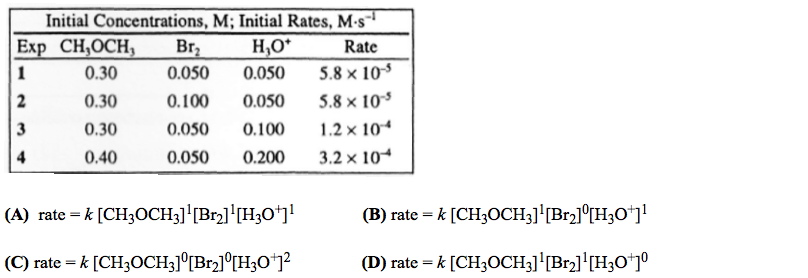# Problem: The reaction between acetone and bromine in acid solution is represented by the equation CH3OCH3(aq) + Br2(aq) + H3O+(aq) → products The tabulated kinetic data were gathered. Based on these data, the experimental rate law is:

###### FREE Expert Solution
100% (188 ratings)
###### Problem Details

The reaction between acetone and bromine in acid solution is represented by the equation

CH3OCH3(aq) + Br2(aq) + H3O+(aq) → products

The tabulated kinetic data were gathered. Based on these data, the experimental rate law is:What scientific concept do you need to know in order to solve this problem?

Our tutors have indicated that to solve this problem you will need to apply the Rate Law concept. You can view video lessons to learn Rate Law. Or if you need more Rate Law practice, you can also practice Rate Law practice problems.

What is the difficulty of this problem?

Our tutors rated the difficulty ofThe reaction between acetone and bromine in acid solution is...as high difficulty.

How long does this problem take to solve?

Our expert Chemistry tutor, Jules took 4 minutes and 42 seconds to solve this problem. You can follow their steps in the video explanation above.

What professor is this problem relevant for?

Based on our data, we think this problem is relevant for Professor Randles' class at UCF.